# Quickly multiply roman mumerals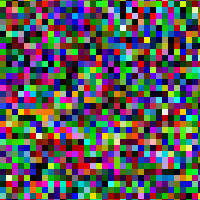A random challenge to myself -- can I think of a way to multiply roman numerals by hand?

I successfully came up with a process I can use quickly with just pencil and paper. The key, for me at least, was to translate into a different set of symbols, which I called "potato bug" numerals. The roman symbols themselves were slowing me down. (When converting from roman numerals, also expand any shorthand so that IV is expanded to IIII and so on). Each hatch mark means a factor of 10.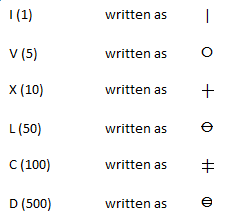and so on. This system is easier to remember and also happens to be quick to write: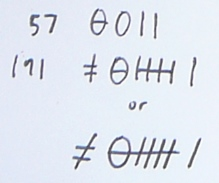Multiplication is now simple. Anything multiplied by I is the number itself. Anything multiplied by ✝ is the number times 10, which is to say: draw another hatch line on everything. When multiplying by 5, one can use the shortcuts in this table: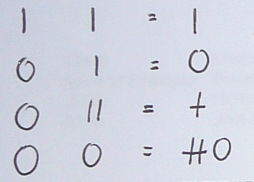To generalize the table - if either or both of the inputs have hatch marks, simply add that many hatch marks on the result. Easy to remember that multiplying by ✝ is just drawing another hatch mark on everything.

One can multiply two numbers by using a "FOIL" like method by the distributive law. To multiply two numbers, take the left-most symbol of the left number, and distribute it across the symbols in the right number. The intermediary results can just be appended in one long string (because there is no place system, a ✝ is 10 wherever it is written). Then take the next symbol in the left number, and again distribute it across the symbols in the right number, and repeat. Finally, simplify the answer by grouping symbols.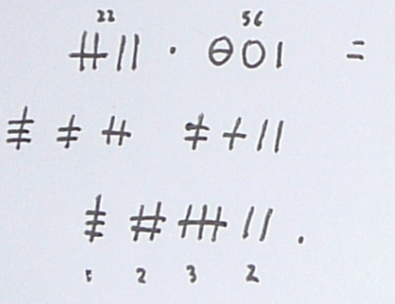There are many operations, but each is trivial and done in a split second. When later "gathering", I would underline to help me remember what I had moved into a group of 5.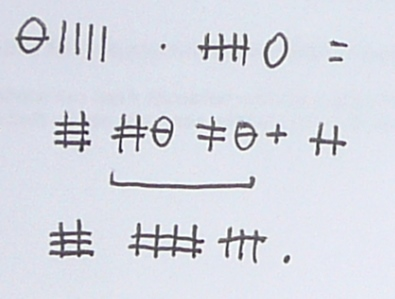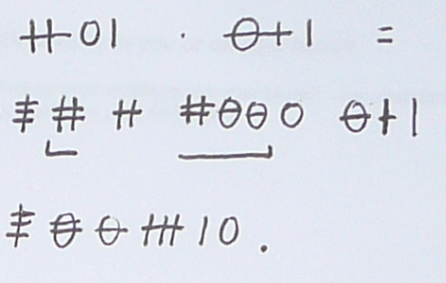So there you have it. Multiplying two digit numbers in seconds with some circles and scratches. Not as slow as you might think.